/

### Measuring the Average Correlation of Stocks in a Universe

1.            The problem

Investors are interested in the average correlation between stocks because it:

(a)    has a potential impact on their ability to add alpha; and

(b)   affects the level of portfolio risk they might be running.

How might we best estimate and measure ‘cross-stock’ correlation? By ‘best’ we mean a suitable combination involving both (i) ease of computation and (ii) relevance to portfolio construction/risk analysis.

2.            The problem

Tierens and Anadu (2004) propose three alternative methods for estimating average stock correlations:

(a) Calculate a full correlation matrix, weighting its elements in line with the weight of the corresponding stocks in the portfolio/index, and excluding correlations between the stock and itself (i.e. the diagonal elements of the correlation matrix)

This involves the following formula, where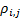is the full correlation matrix, weighting in line with product of the weights in the portfolio of the stocks to which the relevant correlation coefficient relates, but excluding diagonal elements, i.e.: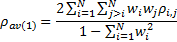Tierens and Anadu argue that this approach yields the most accurate measure of cross-stock correlation, because it uses all the different pair-wise correlations. However, it comes at the expense of computational complexity, as we require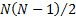correlation coefficients as well as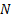portfolio weights, i.e. a total of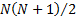inputs. Also, Tierens and Anadu argued that it would in practice be impossible to apply this methodology to compute average market-implied correlations.

(b) Proxy average correlation using only individual stock volatilities and that of the portfolio/index as a whole

We start from the definition of (variance-related) portfolio risk as: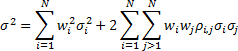where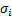is the volatility of the total return of stockand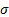is the volatility of the total return of the portfolio.

If we replaceby an average we can back out an implicit calculation for the average cross-sectional correlation as follows: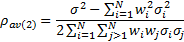This avoids calculating inputs that require pair-wise comparisons; the only inputs we need are single stock volatilities, single stock weights and the volatility of the overall portfolio. The total number of inputs therefore drops to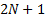. Moreover, it is more practical to apply this methodology to infer the level of average market-implied correlation in the options market, by replacing realised stock and portfolio volatilities by implied stock and portfolio (index) volatilities.

(c) Refine (b) by reference to the ratio of index to average stock volatility

The third method follows the spirit of method (b) and captures the intuition that the volatility of a portfolio is typically lower than the weighted average volatility of the underlying constituents because stocks are less than perfectly correlated. We might therefore, as a rule of thumb, define: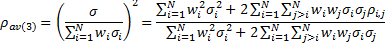3.1          Tierens and Anadu (2004) compared method (b) and method (c) showed that method (b) removes stock variance (as opposed to covariance) whilst method (c) doesn’t but is otherwise similar to (b). A corollary is that as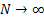(and each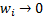) these two proxy methods will converge.

3.2          If we have stocks with identical volatilities then methods (a) and (b) produce the same answer, but method (c) produces a higher answer. However, for stocks with non-identical volatilities then there may be a bias in method (b) too; each pair-wise correlation is weighted by how the product of the volatilities for each of the two stocks in the pair compares to the sum of the volatility products across all pairs of stocks. However, Tierens and Anadu (2004) argue that biases introduced by the simpler methods (b) and (c) appear to be modest in practice for well diversified portfolios/indices.

3.3          This discussion is potentially relevant to the topic of calibrating assumed multivariate normal prior distributions to market implied data, both for instrument pricing of more complicated derivatives and for market implied risk management purposes, see Calibrating Priors to Market Implied Data. However, we find that usually we want to calibrate covariances rather than correlations, since it is the former that drive portfolio risk directly not the latter.  It is thus worth bearing in mind that using as an input to these calibrations an average stock correlation may not be ideal for calibration purposes, except for instruments more directly linked to correlation than to covariance.

References

Tierens, I. and Anadu, M. (2004). Goldman Sachs Quantitative Insights Research Note, 13 April 2004. Goldman Sachs